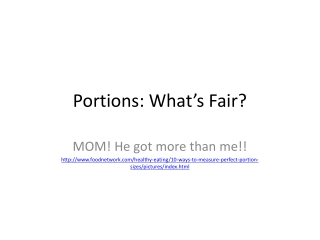DownloadDownload PresentationPortions: What’s Fair?

# Portions: What’s Fair?

Télécharger la présentation## Portions: What’s Fair?

- - - - - - - - - - - - - - - - - - - - - - - - - - - E N D - - - - - - - - - - - - - - - - - - - - - - - - - - -
##### Presentation Transcript

1. Portions: What’s Fair? MOM! He got more than me!! http://www.foodnetwork.com/healthy-eating/10-ways-to-measure-perfect-portion-sizes/pictures/index.html

2. What do we already know about dimensions, portions and ratios? Dimension - A measurement of length in one direction. Examples: width, depth and height are dimensions. Area – the space inside a shape. Portion/Yield – number of servings per dish

3. What affects pizza portion size? • Shape of slice • Size of pan/pizza • Number of slices per pizza • Number of people • Available ingredients • Crust thickness

4. Comparing Pan Dimensions Circular Square/Rectangular

5. Here’s the deal: • We are making pizza tomorrow. • How will you make sure that your group will have the same portion size as the rest of the groups, when you have different pan sizes, i.e. different dimensions of pans?

6. How will we use the dimensions to compare the size of the pizzas, i.e. how will we know who got more (or less, or the same)? • We need to use some math…sigh. • What math concepts/ideas should we use? • Area of circles and rectangles… • Circle formula Rectangle formula • A = A =

7. Circular Pizza Find the area: Area = π •r2 Example: Find the area of a 10” pizza. A = 3.14 x (5 x 5) A = 78.5 sq. inches Square/Rectangular Pizza Find the area: A = length x width A = l x w Example: Find the area of a 10” x 15” pan. A = 10 x 15 A = 150 sq. inches

8. Portion/Yield Portion/yield is: Area divided by number of slices, s. Area ÷ s = Portion size

9. Now complete the table on your worksheet to figure out how many slices are needed to get approximately 20 sq. inches per serving. Circular Area = 78.5 sq. inches A ÷ s = Portion/yield 78.5 ÷ 4 = 19.6 sq. in. Portion size by slice ≈ 20 sq. in. Square/Rectangular Area = 150 sq. in. A ÷ s = Portion/yield 150 ÷ __s_ = 150 ÷ __s_ = 150 ÷ __s_ = Portion size by slice ≈ 20 sq. in.

10. What if it wasn’t a pizza? • Examples: • Cutting fabric into multiple patterns/shapes, how many shapes can you fit in limited yardage? • Making a cake with a cake pan of different dimensions than are called for in the recipe. • Complete the second part of the worksheet, using area and “portion sizes” and conversion factors.

11. So, how do you respond to your sibling or friend who says, “You got a bigger piece of pizza than I did!”? You have to know the dimensions and determine the area, then divide by the number of slices in order to figure out if they are right or not.